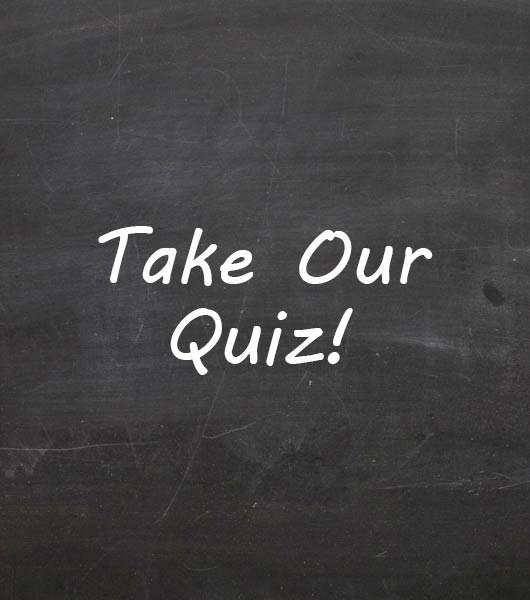Math Minutes Quiz #1

46 Questions | Total Attempts: 111Settings• 1.
Round 0.7643 to the nearest tenth.
• A.

0.76

• B.

0.764

• C.

1

• D.

0.8

• 2.
Round 0.7643 to the nearest hundredth.
• A.

0.76

• B.

0.764

• C.

1

• D.

0.8

• 3.
Round 0.7643 to the nearest thousandth.
• A.

0.76

• B.

0.764

• C.

1

• D.

0.8

• 4.
-8 + -9 =
• A.

17

• B.

-17

• C.

1

• D.

-1

• 5.
-19 + 11 =
• A.

-30

• B.

30

• C.

-8

• D.

8

• 6.
5 + (-12) =
• A.

-7

• B.

7

• C.

-17

• D.

17

• 7.
5 - (-8) =
• A.

3

• B.

-3

• C.

13

• D.

-13

• 8.
-4 - 9 =
• A.

13

• B.

-13

• C.

5

• D.

-5

• 9.
7 • (-9) =
• A.

-2

• B.

-16

• C.

63

• D.

-63

• 10.
-8 • (-4) =
• A.

-32

• B.

32

• C.

-12

• D.

12

• 11.
18 ÷ (-3) =
• A.

15

• B.

-15

• C.

6

• D.

-6

• 12.
-36 ÷ 9 =
• A.

-4

• B.

4

• C.

-27

• D.

27

• 13.
-10 ÷ (-5) =
• A.

2

• B.

-2

• C.

50

• D.

-50

• 14.
0 ÷ (-10) =
• A.

10

• B.

-10

• C.

0

• D.

-0

• 15.
Write 5 • 5 • 5 • 5 using exponents.
• A.

625

• B.

4 to the 5th power

• C.

5 to the 4th power

• D.

20

• 16.
Write x • y • x • y • 5 • 2 • 5 using exponents.
• A.

50 x to the second power • y to the second power

• B.

X to the second power • y to the second power • 5 to the second power • 2

• C.

2 • 5 to the second power • x to the second power • y to the second power

• D.

525 x • x • y • y

• 17.
Write 5,280 in scientific notation.  Show your work.
• A.

528.0 X 10

• B.

5.28 x 10 to the fourth power

• C.

5.28 x 10 to the third power

• D.

52.8 x 10 to the third power

• 18.
3 + 27 ÷ 9 =
• A.

30/9

• B.

6

• C.

3.333...

• D.

21

• 19.
If a = 4 and b = 9, then ab equals:
• A.

13

• B.

36

• C.

49

• D.

94

• 20.
Which of these operations should be completed first when solving an equation?
• A.

+

• B.

X

• C.

÷

• D.

( )

• 21.
Bill's dinner cost \$9.00.  If he wants to leave a 10% tip, how much extra does he leave?
• A.

\$90.00

• B.

\$9.00

• C.

\$1.00

• D.

\$0.90

• 22.
What if Bill wants to leave a 15% tip?  How much extra should he leave?
• A.

\$1.35

• B.

\$0.45

• C.

\$0.90

• D.

\$10.35

• 23.
If Jim scores 6 out of 10 on his math minute, what is his percentage?
• A.

6%

• B.

60%

• C.

600%

• D.

100%

• 24.
Which of the following is not an integer?
• A.

2

• B.

-5

• C.

12/6

• D.

0.5

• 25.
Which of the following is not a whole number?
• A.

12/6

• B.

-7

• C.

0

• D.

13Back to top